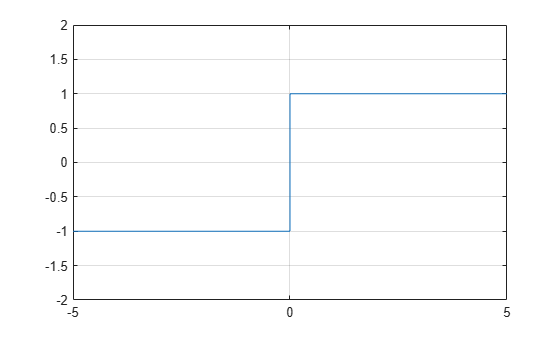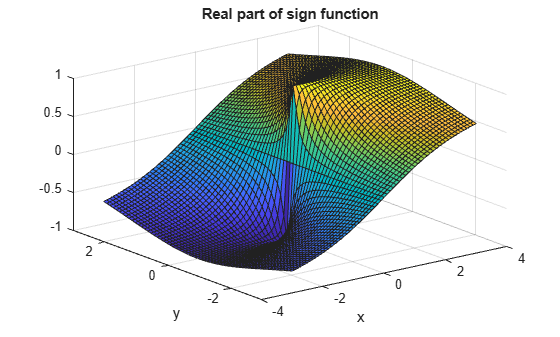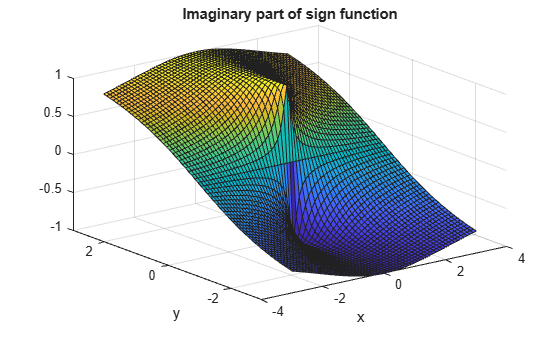Main Content

# sign

Sign function (signum function)

## Syntax

``sign(x)``

## Description

example

```Y = `sign(x)` returns an array `Y` the same size as `x`, where each element of `Y` is: `1` if the corresponding element of `x` is greater than `0`.`0` if the corresponding element of `x` equals `0`.`-1` if the corresponding element of `x` is less than `0`.`x./abs(x)` if `x` is complex. ```

## Examples

collapse all

Find the sign function of a number.

`sign(2)`
```ans = 1 ```

Find the sign function of the values of a vector.

```V = [-11 0 1.5 Inf NaN]; sign(V)```
```ans = 1×5 -1 0 1 1 NaN ```

Find the sign function of the values of a matrix.

```M = magic(3) - 5; sign(M)```
```ans = 3×3 1 -1 1 -1 0 1 -1 1 -1 ```

Find the sign function of a complex number.

```z = 4 - 3*i; sign(z)```
```ans = 0.8000 - 0.6000i ```

Plot the sign function and show its behavior at the zero-crossing. Use `eps` to represent values just above and below `0`.

```x = [-5 -eps(1) 0 eps(1) 5]; y = sign(x); plot(x,y) ylim([-2 2]) grid on```Plot real and imaginary parts of the sign function over $-3 and $-3.

First, create a mesh of values over `-3 < x < 3` and `-3 < y < 3` using `meshgrid`. Then create complex numbers from these values using `z = x + 1i*y`.

```v = -3:0.1:3; [x, y] = meshgrid(v); z = x + 1i*y;```

Find the real and imaginary parts of the sign function of `z`.

```s = sign(z); re = real(s); im = imag(s);```

Plot the real and imaginary parts.

```surf(x,y,re) title('Real part of sign function') xlabel('x') ylabel('y')``````figure(2) surf(x,y,im) title('Imaginary part of sign function') xlabel('x') ylabel('y')```## Input Arguments

collapse all

Input, specified as a scalar, vector, matrix, or multidimensional array.

If an element of `x` is `NaN`, then `sign` returns `NaN` in the corresponding element of the output.

Data Types: `single` | `double` | `int8` | `int16` | `int32` | `int64` | `uint8` | `uint16` | `uint32` | `uint64` | `logical` | `duration`
Complex Number Support: Yes

## See Also

Introduced before R2006a

Download ebook